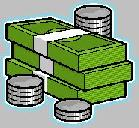Financial Terms Phillips Curve

# Definition of Phillips Curve## Phillips Curve

Relationship between inflation and unemployment.

# Related Terms:

## Aggregate Demand Curve

Combinations of the price level and income for which the goods and services market is in equilibrium, or for which both the goods and services market and the money market are in equilibrium.

## Aggregate Expenditure Curve

Aggregate demand for goods and services drawn as a function of the level of national income.

## Aggregate Supply Curve

Combinations of price level and income for which the labor market is in equilibrium. The short-run aggregate supply curve incorporates information and price/wage inflexibilities in the labor market, whereas the long-run aggregate supply curve does not.

## Discount curve

The curve of discount rates vs. maturity dates for bonds.

## Flattening of the yield curve

A change in the yield curve where the spread between the yield on a long-term
and short-term Treasury has decreased. Compare steepening of the yield curve and butterfly shift.

## Indifference curve

The graphical expression of a utility function, where the horizontal axis measures risk and
the vertical axis measures expected return. The curve connects all portfolios with the same utilities according
to g and s .

## J-curve

Theory that says a country's trade deficit will initially worsen after its currency depreciates because
higher prices on foreign imports will more than offset the reduced volume of imports in the short-run.## Laffer Curve

curve showing how tax receipts vary with the tax rate.

## learning curve

a model that helps predict how labor time
will decrease as people become more experienced at performing
a task and eliminate the inefficiencies associated
with unfamiliarity

## Non-parallel shift in the yield curve

A shift in the yield curve in which yields do not change by the same
number of basis points for every maturity. Related: Parallel shift in the yield curve.

## Par yield curve

The yield curve of bonds selling at par, or face, value.

## Parallel shift in the yield curve

A shift in the yield curve in which the change in the yield on all maturities is
the same number of basis points. In other words, if the 3 month T-bill increases 100 basis points (one
percent), then the 6 month, 1 year, 5 year, 10 year, 20 year, and 30 year rates increase by 100 basis points as
well.
Related: Non-parallel shift in the yield curve.

## Riding the yield curve

Buying long-term bonds in anticipation of capital gains as yields fall with the
declining maturity of the bonds.

See Zero curve.

## Spot rate curve

The graphical depiction of the relationship between the spot rates and maturity.

## Steepening of the yield curve

A change in the yield curve where the spread between the yield on a long-term
and short-term Treasury has increased. Compare flattening of the yield curve and butterfly shift.## Stopping curve

A curve showing the refunding rates for different points in time at which the expected value
of refunding immediately equals the expected value of waiting to refund.

## Stopping curve refunding rate

A refunding rate that falls on the stopping curve.

## Theoretical spot rate curve

A curve derived from theoretical considerations as applied to the yields of
actually traded Treasury debt securities because there are no zero-coupon Treasury debt issues with a maturity
greater than one year. Like the yield curve, this is a graphical depiction of the term structure of interest rates.

## Yield curve

The graphical depiction of the relationship between the yield on bonds of the same credit quality
but different maturities. Related: Term structure of interest rates. Harvey (1991) finds that the inversions of
the yield curve (short-term rates greater than long term rates) have preceded the last five U.S. recessions. The
yield curve can accurately forecast the turning points of the business cycle.

## Yield Curve

A graphical representation of the level of interest rates for
securities of differing maturities at a specific point of time

## Yield curve

Graph of yields (vertical axis) of a particular type of security
versus the time to maturity (horizontal axis). This curve usually slopes
securities that have a longer time to maturity. The benchmark yield curve is
for U.S. Treasury securities with maturities ranging from three months to 30
years. See Term structure.

## yield curve

Graph of the relationship between time to maturity and yield to maturity.

## Yield curve

A graph showing how the yield on bonds varies with time to maturity.

## Yield curve option-pricing models

Models that can incorporate different volatility assumptions along the
yield curve, such as the Black-Derman-Toy model. Also called arbitrage-free option-pricing models.

## Yield curve strategies

Positioning a portfolio to capitalize on expected changes in the shape of the Treasury yield curve.

## Zero curve, zero-coupon yield curve

A yield curve for zero-coupon bonds;
zero rates versus maturity dates. Since the maturity and duration (Macaulay
duration) are identical for zeros, the zero curve is a pure depiction of supply/
demand conditions for loanable funds across a continuum of durations and
maturities. Also known as spot curve or spot yield curve.